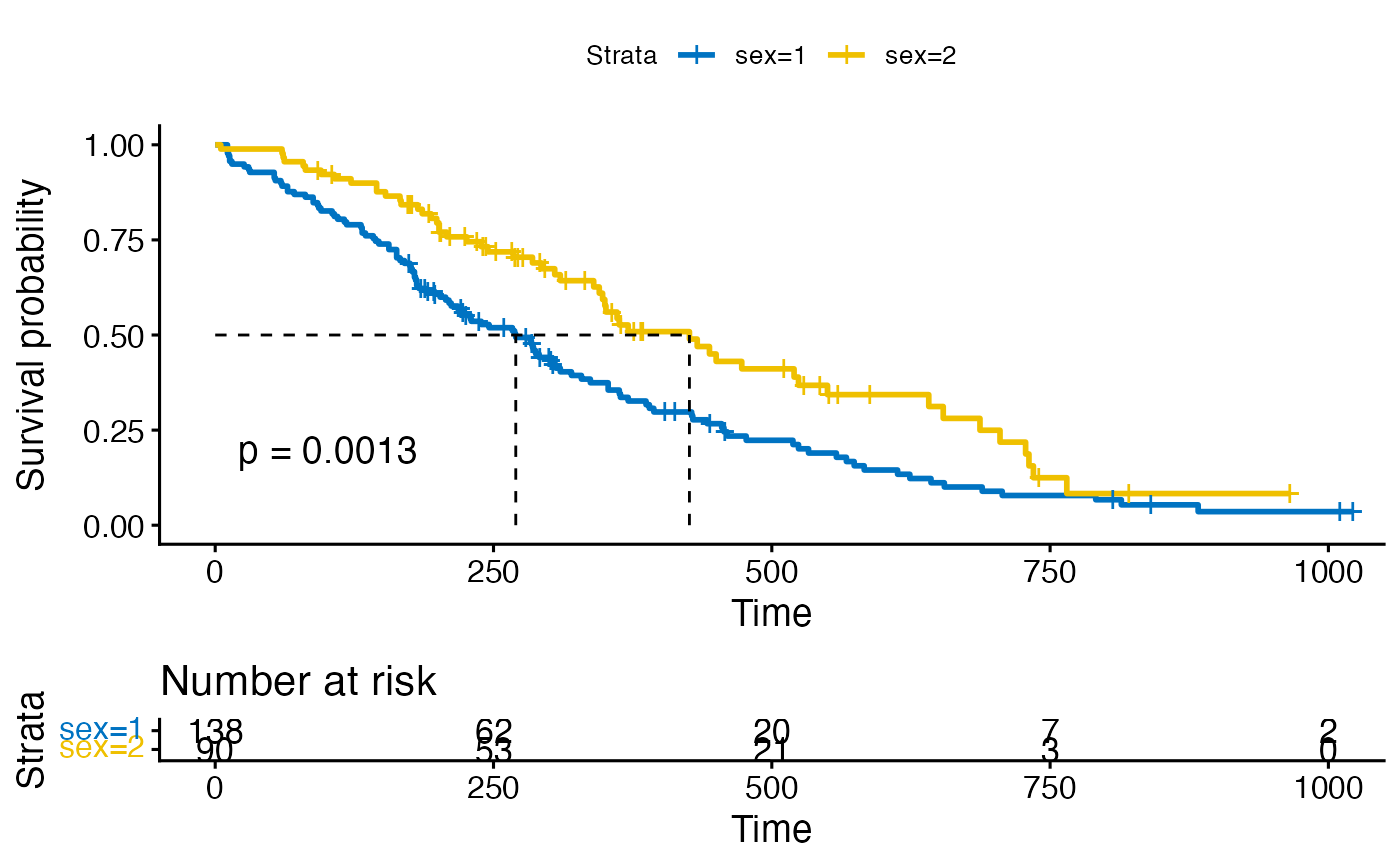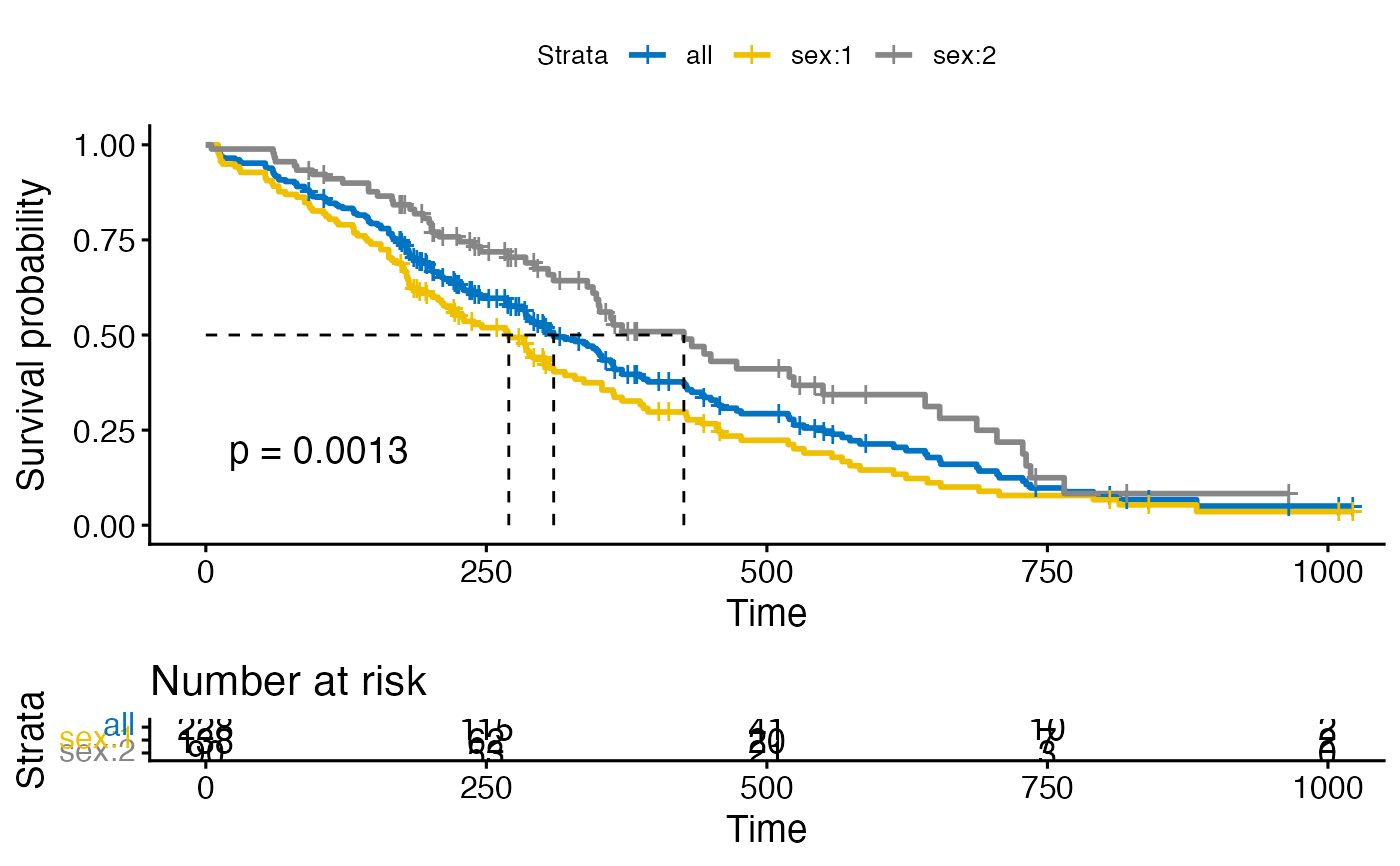Add survival curves of pooled patients onto the main plot stratified by grouping variables.

ggsurvplot_add_all(
fit,
data,
legend.title = "Strata",
legend.labs = NULL,
pval = FALSE,
...
)

## Arguments

fit an object of class survfit. a dataset used to fit survival curves. If not supplied then data will be extracted from 'fit' object. legend title. character vector specifying legend labels. Used to replace the names of the strata from the fit. Should be given in the same order as those strata. logical value, a numeric or a string. If logical and TRUE, the p-value is added on the plot. If numeric, than the computet p-value is substituted with the one passed with this parameter. If character, then the customized string appears on the plot. See examples - Example 3. other arguments passed to the ggsurvplot() function.

## Value

Return a ggsurvplot.

ggsurvplot

## Examples

library(survival)

# Fit survival curves
fit <- surv_fit(Surv(time, status) ~ sex, data = lung)

# Visualize survival curves
ggsurvplot(fit, data = lung,
risk.table = TRUE, pval = TRUE,
surv.median.line = "hv", palette = "jco")# Add survival curves of pooled patients (Null model)
# Use add.all = TRUE option
ggsurvplot(fit, data = lung,
risk.table = TRUE, pval = TRUE,
surv.median.line = "hv", palette = "jco",
add.all = TRUE)